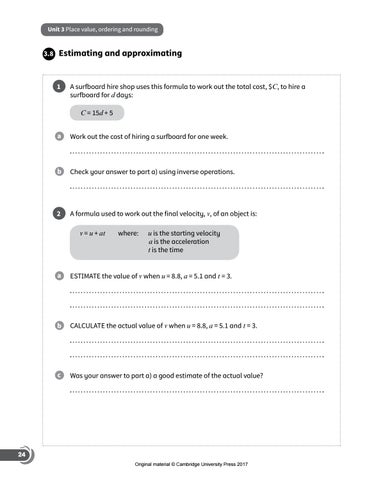# Preview Cambridge Checkpoint Mathematics Challenge Book 7Unit 3 Place value, ordering and rounding

3.8

Estimating and approximating

1

A surfboard hire shop uses this formula to work out the total cost, \$C, to hire a surfboard for d days: C = 15d + 5

a Work out the cost of hiring a surfboard for one week.  b Check your answer to part a) using inverse operations.

2

v, of an object is: A formula used to work out the final velocity, v = u + at where: u is the starting velocity a is the acceleration t is the time

a Estimate the value of v when u = 8.8, a = 5.1 and t = 3.  b Calculate the actual value of v when u = 8.8, a = 5.1 and t = 3. c Was your answer to part a) a good estimate of the actual value?

24 Original material © Cambridge University Press 2017